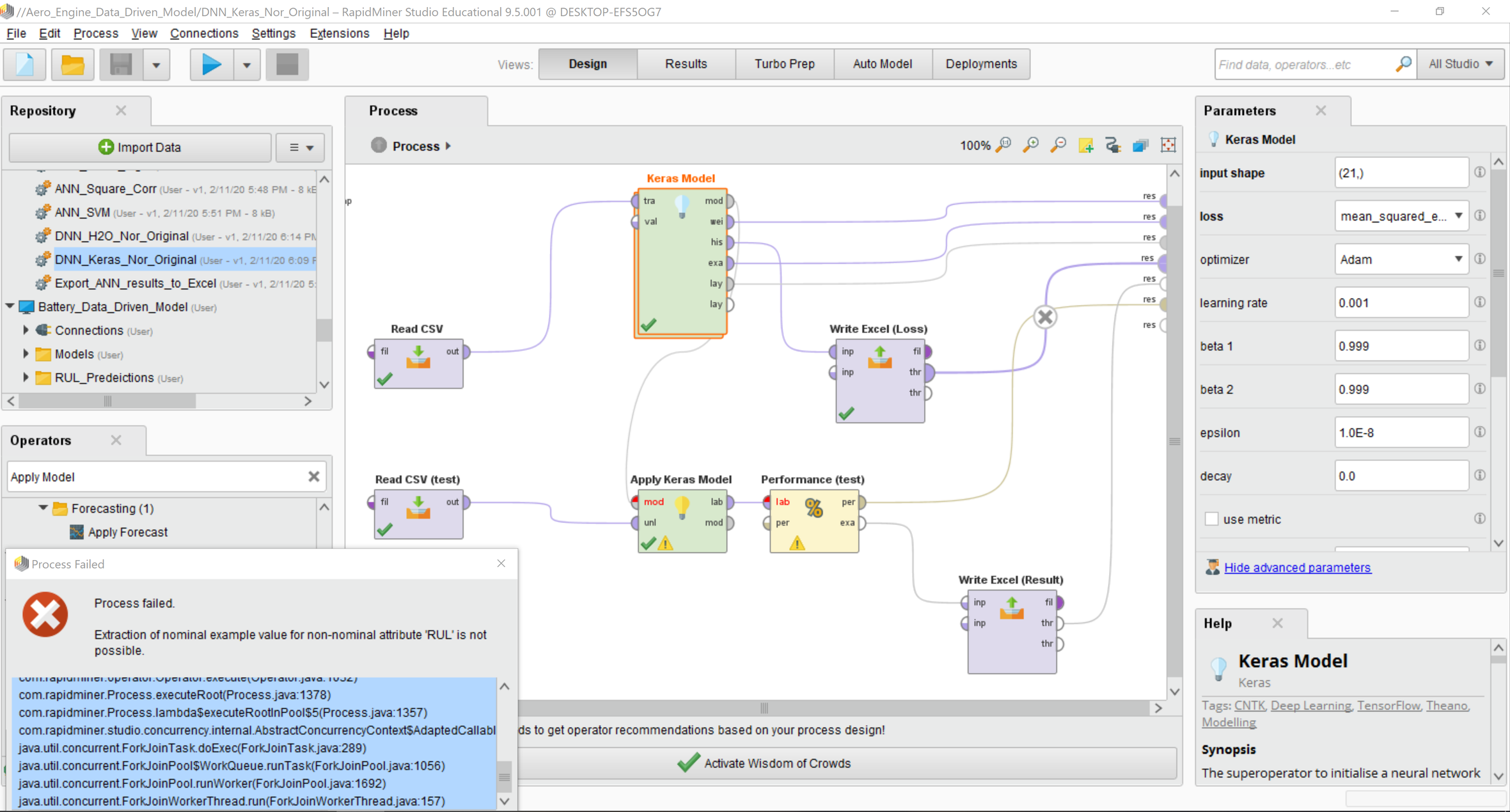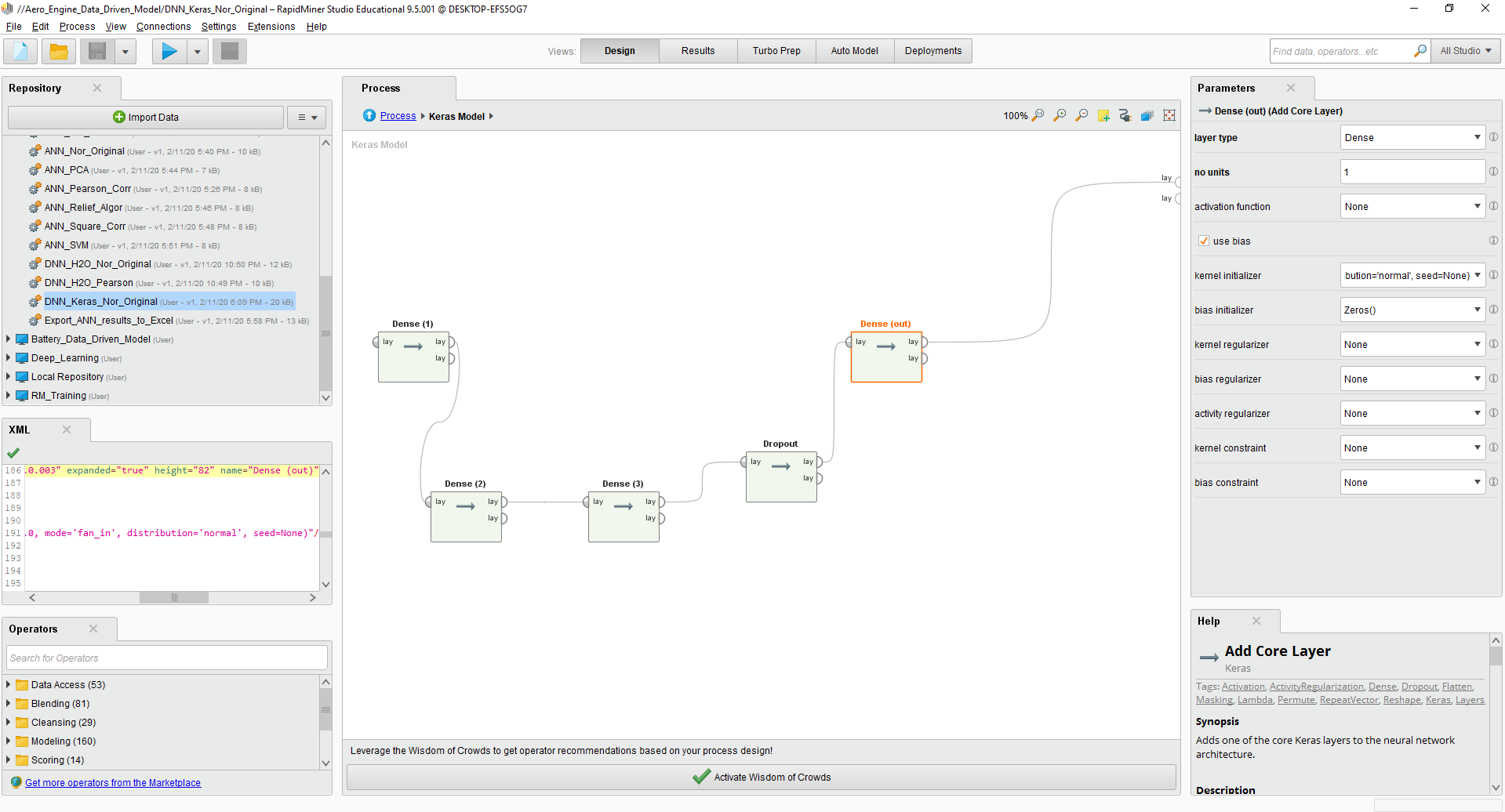# Bug message when running Keras: Extraction of nominal example value for non-nominal attribute 'RUL'

Member Posts: 3Contributor I
edited February 2020 in Help
Hello, I have the following bug below when I tried to run Keras (Deep Neural Net 3 Dense layer with 1 Dropout layer). I've also attached the screenshot of my process. Note that I tried the same process with the different datasets but got no issue. Both datasets predict the numerical value (the label is number, not category). If anyone knows it's a bug or I did something wrong ... please ... please help.

Thank you!====================================================================================

Exception: com.rapidminer.example.AttributeTypeException
Message: Extraction of nominal example value for non-nominal attribute 'RUL' is not possible.
Stack trace:

com.rapidminer.example.Example.getNominalValue(Example.java:98)
com.rapidminer.operator.performance.SimpleCriterion.countExample(SimpleCriterion.java:93)
com.rapidminer.operator.performance.AbstractPerformanceEvaluator.evaluate(AbstractPerformanceEvaluator.java:470)
com.rapidminer.operator.performance.AbstractPerformanceEvaluator.evaluate(AbstractPerformanceEvaluator.java:393)
com.rapidminer.operator.performance.AbstractPerformanceEvaluator.doWork(AbstractPerformanceEvaluator.java:256)
com.rapidminer.operator.Operator.execute(Operator.java:1032)
com.rapidminer.operator.execution.SimpleUnitExecutor.execute(SimpleUnitExecutor.java:77)
com.rapidminer.operator.ExecutionUnit\$2.run(ExecutionUnit.java:812)
com.rapidminer.operator.ExecutionUnit\$2.run(ExecutionUnit.java:807)
java.security.AccessController.doPrivileged(Native Method)
com.rapidminer.operator.ExecutionUnit.execute(ExecutionUnit.java:807)
com.rapidminer.operator.OperatorChain.doWork(OperatorChain.java:423)
com.rapidminer.operator.Operator.execute(Operator.java:1032)
com.rapidminer.Process.executeRoot(Process.java:1378)
com.rapidminer.Process.lambda\$executeRootInPool\$5(Process.java:1357)
java.util.concurrent.ForkJoinPool.runWorker(ForkJoinPool.java:1692)

• Moderator, RapidMiner Certified Analyst, Member, University Professor Posts: 568Unicorn
Hello pxkst970,

Do you mind to share with us the actual XML of your process and the code that is failing? I'm pretty sure it's an issue with data cleaning, but better safe than sorry. If you can't publish it, you may use the private messaging for us to check.

All the best,

Rodrigo.
• Member Posts: 3Contributor I
Hello rfuentealba
Sure, no problem. I can share the actual XML with you below.
I suspected that for some reason, the Keras layer transformed my label (or prediction attribute) into nominal data. This is the regression problem and my label is numerical data. There is certainly no problem with data cleaning because I use this same dataset to do one layer ANN (Artificial Neural Net) in RapidMiner before I tried Keras. The problem occurred when applying the model from Keras into the test dataset.

I've also attached the layer in the Keras process for you as well. The problem might come from the last layer. As I understand the last layer should act as an output layer and should be a dense layer with no activation function, right? Please help to correct me if I'm wrong.

=========================================================================
<?xml version="1.0" encoding="UTF-8"?><process version="9.5.001">
<context>
<input/>
<output/>
<macros/>
</context>
<operator activated="true" class="process" compatibility="9.4.000" expanded="true" name="Process">
<parameter key="logverbosity" value="init"/>
<parameter key="random_seed" value="2001"/>
<parameter key="send_mail" value="never"/>
<parameter key="process_duration_for_mail" value="30"/>
<parameter key="encoding" value="SYSTEM"/>
<process expanded="true">
<parameter key="csv_file" value="C:\Users\User\Desktop\NDSU\Research\6. Data\Aero_Engine\Experiment_FD002\1_Results_Final\Selected_Input_Final\Aero_Engine_1_FD002_z-normailized_Ori.csv"/>
<parameter key="column_separators" value=","/>
<parameter key="trim_lines" value="false"/>
<parameter key="use_quotes" value="true"/>
<parameter key="quotes_character" value="&quot;"/>
<parameter key="escape_character" value="\"/>
<parameter key="comment_characters" value="#"/>
<parameter key="starting_row" value="1"/>
<parameter key="parse_numbers" value="true"/>
<parameter key="decimal_character" value="."/>
<parameter key="grouped_digits" value="false"/>
<parameter key="grouping_character" value=","/>
<parameter key="infinity_representation" value=""/>
<parameter key="date_format" value=""/>
<parameter key="first_row_as_names" value="true"/>
<list key="annotations">
<parameter key="0" value="Name"/>
</list>
<parameter key="time_zone" value="SYSTEM"/>
<parameter key="locale" value="English (United States)"/>
<parameter key="encoding" value="UTF-8"/>
<list key="data_set_meta_data_information">
<parameter key="0" value="T2.true.real.attribute"/>
<parameter key="1" value="T24.true.real.attribute"/>
<parameter key="2" value="T30.true.real.attribute"/>
<parameter key="3" value="T50.true.real.attribute"/>
<parameter key="4" value="P2.true.real.attribute"/>
<parameter key="5" value="P15.true.real.attribute"/>
<parameter key="6" value="P30.true.real.attribute"/>
<parameter key="7" value="Nf.true.real.attribute"/>
<parameter key="8" value="Nc.true.real.attribute"/>
<parameter key="9" value="epr.true.real.attribute"/>
<parameter key="10" value="Ps30.true.real.attribute"/>
<parameter key="11" value="phi.true.real.attribute"/>
<parameter key="12" value="NRF.true.real.attribute"/>
<parameter key="13" value="NRc.true.real.attribute"/>
<parameter key="14" value="BPR.true.real.attribute"/>
<parameter key="15" value="farB.true.real.attribute"/>
<parameter key="16" value="htBleed.true.real.attribute"/>
<parameter key="17" value="Nf_dmd.true.real.attribute"/>
<parameter key="18" value="PCNfR_dmd.true.real.attribute"/>
<parameter key="19" value="W31.true.real.attribute"/>
<parameter key="20" value="W32.true.real.attribute"/>
<parameter key="21" value="time_cycle.true.integer.id"/>
<parameter key="22" value="RUL.true.real.label"/>
</list>
<parameter key="datamanagement" value="double_array"/>
<parameter key="data_management" value="auto"/>
</operator>
<operator activated="true" class="keras:sequential" compatibility="1.0.003" expanded="true" height="166" name="Keras Model" width="90" x="313" y="34">
<parameter key="input shape" value="(21,)"/>
<parameter key="loss" value="mean_squared_error"/>
<parameter key="learning rate" value="0.001"/>
<parameter key="momentum" value="0.0"/>
<parameter key="rho" value="0.9"/>
<parameter key="beta 1" value="0.999"/>
<parameter key="beta 2" value="0.999"/>
<parameter key="epsilon" value="1.0E-8"/>
<parameter key="decay" value="0.0"/>
<parameter key="schedule decay" value="0.004"/>
<parameter key="Nesterov" value="false"/>
<parameter key="use metric" value="false"/>
<enumeration key="metric"/>
<parameter key="epochs" value="526"/>
<parameter key="batch size" value="1"/>
<enumeration key="callbacks">
<parameter key="callbacks" value="TensorBoard(log_dir='./logs', histogram_freq=0, write_graph=True, write_images=False, embeddings_freq=0, embeddings_layer_names=None, embeddings_metadata=None)"/>
</enumeration>
<parameter key="verbose" value="1"/>
<parameter key="validation split" value="0.0"/>
<parameter key="shuffle" value="false"/>
<parameter key="fix seed" value="false"/>
<parameter key="random seed" value="0"/>
<process expanded="true">
<operator activated="true" class="keras:core_layer" compatibility="1.0.003" expanded="true" height="82" name="Dense (1)" width="90" x="45" y="238">
<parameter key="layer_type" value="Dense"/>
<parameter key="no_units" value="12"/>
<parameter key="activation_function" value="'relu'"/>
<parameter key="use_bias" value="true"/>
<parameter key="kernel_initializer" value="VarianceScaling(scale=1.0, mode='fan_in', distribution='normal', seed=None)"/>
<parameter key="bias_initializer" value="Zeros()"/>
<parameter key="kernel_regularizer" value="None"/>
<parameter key="bias_regularizer" value="None"/>
<parameter key="activity_regularizer" value="None"/>
<parameter key="kernel_constraint" value="None"/>
<parameter key="bias_constraint" value="None"/>
<parameter key="rate" value="0.1"/>
<parameter key="noise_shape" value="None"/>
<parameter key="seed" value="None"/>
<parameter key="target_shape" value="(1, 1)"/>
<parameter key="dims" value="1.1"/>
<parameter key="repetition_factor" value="2"/>
<parameter key="function" value="None"/>
<parameter key="l1" value="0.0"/>
<parameter key="l2" value="0.0"/>
</operator>
<operator activated="true" class="keras:core_layer" compatibility="1.0.003" expanded="true" height="82" name="Dense (2)" width="90" x="112" y="442">
<parameter key="layer_type" value="Dense"/>
<parameter key="no_units" value="12"/>
<parameter key="activation_function" value="'relu'"/>
<parameter key="use_bias" value="true"/>
<parameter key="kernel_initializer" value="VarianceScaling(scale=1.0, mode='fan_in', distribution='normal', seed=None)"/>
<parameter key="bias_initializer" value="Zeros()"/>
<parameter key="kernel_regularizer" value="None"/>
<parameter key="bias_regularizer" value="None"/>
<parameter key="activity_regularizer" value="None"/>
<parameter key="kernel_constraint" value="None"/>
<parameter key="bias_constraint" value="None"/>
<parameter key="rate" value="0.1"/>
<parameter key="noise_shape" value="None"/>
<parameter key="seed" value="None"/>
<parameter key="target_shape" value="(1, 1)"/>
<parameter key="dims" value="1.1"/>
<parameter key="repetition_factor" value="2"/>
<parameter key="function" value="None"/>
<parameter key="l1" value="0.0"/>
<parameter key="l2" value="0.0"/>
</operator>
<operator activated="true" class="keras:core_layer" compatibility="1.0.003" expanded="true" height="82" name="Dense (3)" width="90" x="313" y="442">
<parameter key="layer_type" value="Dense"/>
<parameter key="no_units" value="12"/>
<parameter key="activation_function" value="'relu'"/>
<parameter key="use_bias" value="true"/>
<parameter key="kernel_initializer" value="VarianceScaling(scale=1.0, mode='fan_in', distribution='normal', seed=None)"/>
<parameter key="bias_initializer" value="Zeros()"/>
<parameter key="kernel_regularizer" value="None"/>
<parameter key="bias_regularizer" value="None"/>
<parameter key="activity_regularizer" value="None"/>
<parameter key="kernel_constraint" value="None"/>
<parameter key="bias_constraint" value="None"/>
<parameter key="rate" value="0.1"/>
<parameter key="noise_shape" value="None"/>
<parameter key="seed" value="None"/>
<parameter key="target_shape" value="(1, 1)"/>
<parameter key="dims" value="1.1"/>
<parameter key="repetition_factor" value="2"/>
<parameter key="function" value="None"/>
<parameter key="l1" value="0.0"/>
<parameter key="l2" value="0.0"/>
</operator>
<operator activated="true" class="keras:core_layer" compatibility="1.0.003" expanded="true" height="82" name="Dropout" width="90" x="514" y="391">
<parameter key="layer_type" value="Dropout"/>
<parameter key="no_units" value="1"/>
<parameter key="activation_function" value="None"/>
<parameter key="use_bias" value="true"/>
<parameter key="kernel_initializer" value="glorot_uniform(seed=None)"/>
<parameter key="bias_initializer" value="Zeros()"/>
<parameter key="kernel_regularizer" value="None"/>
<parameter key="bias_regularizer" value="None"/>
<parameter key="activity_regularizer" value="None"/>
<parameter key="kernel_constraint" value="None"/>
<parameter key="bias_constraint" value="None"/>
<parameter key="rate" value="0.25"/>
<parameter key="noise_shape" value="None"/>
<parameter key="seed" value="None"/>
<parameter key="target_shape" value=""/>
<parameter key="dims" value="1.1"/>
<parameter key="repetition_factor" value="1"/>
<parameter key="function" value="None"/>
<parameter key="l1" value="0.0"/>
<parameter key="l2" value="0.0"/>
</operator>
<operator activated="true" class="keras:core_layer" compatibility="1.0.003" expanded="true" height="82" name="Dense (out)" width="90" x="648" y="238">
<parameter key="layer_type" value="Dense"/>
<parameter key="no_units" value="1"/>
<parameter key="activation_function" value="None"/>
<parameter key="use_bias" value="true"/>
<parameter key="kernel_initializer" value="VarianceScaling(scale=1.0, mode='fan_in', distribution='normal', seed=None)"/>
<parameter key="bias_initializer" value="Zeros()"/>
<parameter key="kernel_regularizer" value="None"/>
<parameter key="bias_regularizer" value="None"/>
<parameter key="activity_regularizer" value="None"/>
<parameter key="kernel_constraint" value="None"/>
<parameter key="bias_constraint" value="None"/>
<parameter key="rate" value="0.1"/>
<parameter key="noise_shape" value="None"/>
<parameter key="seed" value="None"/>
<parameter key="target_shape" value="1.1"/>
<parameter key="dims" value="1.1"/>
<parameter key="repetition_factor" value="2"/>
<parameter key="function" value="None"/>
<parameter key="l1" value="0.0"/>
<parameter key="l2" value="0.0"/>
</operator>
<connect from_op="Dense (1)" from_port="layers 1" to_op="Dense (2)" to_port="layers"/>
<connect from_op="Dense (2)" from_port="layers 1" to_op="Dense (3)" to_port="layers"/>
<connect from_op="Dense (3)" from_port="layers 1" to_op="Dropout" to_port="layers"/>
<connect from_op="Dropout" from_port="layers 1" to_op="Dense (out)" to_port="layers"/>
<connect from_op="Dense (out)" from_port="layers 1" to_port="layers 1"/>
<portSpacing port="sink_layers 1" spacing="0"/>
<portSpacing port="sink_layers 2" spacing="0"/>
</process>
</operator>
<operator activated="true" class="write_excel" compatibility="9.2.001" expanded="true" height="103" name="Write Excel (Loss)" width="90" x="514" y="187">
<parameter key="excel_file" value="C:\Users\User\Desktop\NDSU\Research\6. Data\Aero_Engine\Experiment_FD002\1_Results_Final_Latest\Aero_Keras_DNN_Loss_Function.xlsx"/>
<parameter key="file_format" value="xlsx"/>
<enumeration key="sheet_names"/>
<parameter key="sheet_name" value="RapidMiner Data"/>
<parameter key="date_format" value="yyyy-MM-dd HH:mm:ss"/>
<parameter key="number_format" value="#.0"/>
<parameter key="encoding" value="SYSTEM"/>
</operator>
<operator activated="true" class="read_csv" compatibility="9.5.001" expanded="true" height="68" name="Read CSV (test)" width="90" x="45" y="340">
<parameter key="csv_file" value="C:\Users\User\Desktop\NDSU\Research\6. Data\Aero_Engine\Experiment_FD002\1_Results_Final\Selected_Input_Final\Test_Data_Engine-46_to_60_z-normalized_Ori.csv"/>
<parameter key="column_separators" value=","/>
<parameter key="trim_lines" value="false"/>
<parameter key="use_quotes" value="true"/>
<parameter key="quotes_character" value="&quot;"/>
<parameter key="escape_character" value="\"/>
<parameter key="comment_characters" value="#"/>
<parameter key="starting_row" value="1"/>
<parameter key="parse_numbers" value="true"/>
<parameter key="decimal_character" value="."/>
<parameter key="grouped_digits" value="false"/>
<parameter key="grouping_character" value=","/>
<parameter key="infinity_representation" value=""/>
<parameter key="date_format" value=""/>
<parameter key="first_row_as_names" value="true"/>
<list key="annotations">
<parameter key="0" value="Name"/>
</list>
<parameter key="time_zone" value="SYSTEM"/>
<parameter key="locale" value="English (United States)"/>
<parameter key="encoding" value="UTF-8"/>
<list key="data_set_meta_data_information">
<parameter key="0" value="T2.true.real.attribute"/>
<parameter key="1" value="T24.true.real.attribute"/>
<parameter key="2" value="T30.true.real.attribute"/>
<parameter key="3" value="T50.true.real.attribute"/>
<parameter key="4" value="P2.true.real.attribute"/>
<parameter key="5" value="P15.true.real.attribute"/>
<parameter key="6" value="P30.true.real.attribute"/>
<parameter key="7" value="Nf.true.real.attribute"/>
<parameter key="8" value="Nc.true.real.attribute"/>
<parameter key="9" value="epr.true.real.attribute"/>
<parameter key="10" value="Ps30.true.real.attribute"/>
<parameter key="11" value="phi.true.real.attribute"/>
<parameter key="12" value="NRF.true.real.attribute"/>
<parameter key="13" value="NRc.true.real.attribute"/>
<parameter key="14" value="BPR.true.real.attribute"/>
<parameter key="15" value="farB.true.real.attribute"/>
<parameter key="16" value="htBleed.true.real.attribute"/>
<parameter key="17" value="Nf_dmd.true.real.attribute"/>
<parameter key="18" value="PCNfR_dmd.true.real.attribute"/>
<parameter key="19" value="W31.true.real.attribute"/>
<parameter key="20" value="W32.true.real.attribute"/>
<parameter key="21" value="time_cycle.true.integer.id"/>
<parameter key="22" value="RUL.true.real.label"/>
</list>
<parameter key="datamanagement" value="double_array"/>
<parameter key="data_management" value="auto"/>
</operator>
<operator activated="true" class="keras:apply" compatibility="1.0.003" expanded="true" height="82" name="Apply Keras Model" width="90" x="313" y="340">
<parameter key="batch_size" value="1"/>
<parameter key="verbose" value="0"/>
</operator>
<operator activated="true" class="performance_regression" compatibility="9.5.001" expanded="true" height="82" name="Performance (test)" width="90" x="447" y="340">
<parameter key="main_criterion" value="root_mean_squared_error"/>
<parameter key="root_mean_squared_error" value="true"/>
<parameter key="absolute_error" value="false"/>
<parameter key="relative_error" value="false"/>
<parameter key="relative_error_lenient" value="false"/>
<parameter key="relative_error_strict" value="false"/>
<parameter key="normalized_absolute_error" value="false"/>
<parameter key="root_relative_squared_error" value="false"/>
<parameter key="squared_error" value="false"/>
<parameter key="correlation" value="false"/>
<parameter key="squared_correlation" value="false"/>
<parameter key="prediction_average" value="false"/>
<parameter key="spearman_rho" value="false"/>
<parameter key="kendall_tau" value="false"/>
<parameter key="skip_undefined_labels" value="true"/>
<parameter key="use_example_weights" value="true"/>
</operator>
<operator activated="true" class="write_excel" compatibility="9.2.001" expanded="true" height="103" name="Write Excel (Result)" width="90" x="648" y="442">
<parameter key="excel_file" value="C:\Users\User\Desktop\NDSU\Research\6. Data\Aero_Engine\Experiment_FD002\1_Results_Final_Latest\Aero_Keras_DNN_Result.xlsx"/>
<parameter key="file_format" value="xlsx"/>
<enumeration key="sheet_names"/>
<parameter key="sheet_name" value="RapidMiner Data"/>
<parameter key="date_format" value="yyyy-MM-dd HH:mm:ss"/>
<parameter key="number_format" value="#.0"/>
<parameter key="encoding" value="SYSTEM"/>
</operator>
<connect from_op="Read CSV" from_port="output" to_op="Keras Model" to_port="training set"/>
<connect from_op="Keras Model" from_port="model" to_op="Apply Keras Model" to_port="model"/>
<connect from_op="Keras Model" from_port="weights" to_port="result 1"/>
<connect from_op="Keras Model" from_port="history" to_op="Write Excel (Loss)" to_port="input"/>
<connect from_op="Keras Model" from_port="example set" to_port="result 2"/>
<connect from_op="Keras Model" from_port="layers 1" to_port="result 3"/>
<connect from_op="Write Excel (Loss)" from_port="through" to_port="result 4"/>
<connect from_op="Read CSV (test)" from_port="output" to_op="Apply Keras Model" to_port="unlabelled data"/>
<connect from_op="Apply Keras Model" from_port="labelled data" to_op="Performance (test)" to_port="labelled data"/>
<connect from_op="Performance (test)" from_port="performance" to_port="result 6"/>
<connect from_op="Performance (test)" from_port="example set" to_op="Write Excel (Result)" to_port="input"/>
<connect from_op="Write Excel (Result)" from_port="through" to_port="result 5"/>
<portSpacing port="source_input 1" spacing="0"/>
<portSpacing port="sink_result 1" spacing="0"/>
<portSpacing port="sink_result 2" spacing="0"/>
<portSpacing port="sink_result 3" spacing="0"/>
<portSpacing port="sink_result 4" spacing="0"/>
<portSpacing port="sink_result 5" spacing="0"/>
<portSpacing port="sink_result 6" spacing="0"/>
<portSpacing port="sink_result 7" spacing="0"/>
</process>
</operator>
</process>